# Arrays in C#

## Arrays in C# with Examples

In this article, I will discuss Arrays in C# with Examples. It is one of the most important concepts in programming languages. Arrays are there from our traditional programming languages such as C, and C++ and are also available in C#. As part of this article, we are going to discuss the following pointers.

1. Why do we need arrays in programming?
2. What is an Array in C#?
3. Types of Arrays in C#.
4. How to Create and Initialize an Array?
5. How to access the Array Elements in C#?
6. Understanding the memory representation of the array in C#.
7. One Dimensional Array in C# with Examples
8. What is the difference between for loop and for each loop in C# to access array values?
9. What is the Array class in C#?
10. Understanding the Array class methods and properties.
11. What is an Implicitly Type Array in C#?
##### Why do we need Arrays in Programming?

As we know a primitive type variable such as int, double, bool, etc. can hold only a single value at any given point in time. For example, int Number = 10;. Here the variable Number holds a value of 10. As per your business requirement, if you want to store 100 integer values, then you need to create 100 integer variables in your program which is not a good programming approach as it will take lots of time as well as your code becomes bigger. We can overcome this problem by using the concept called Arrays, and Arrays are not new in C#, they are existing in other programming languages such as C, C++, Java, etc. Using Arrays, instead of creating 100 variables to hold 100 values, we can create a single variable which can hold 100 values.

##### What is an Array in C#?

The array is defined as a collection of similar data elements. If you have some sets of integers, and some sets of floats, you can group them under one name as an array. So, in simple words, we can define an array as a collection of similar types of values that are stored in a contiguous memory location under a single name.

An array is a kind of sequential data structure, which is used to store the collection of items of the same type. I am sure you did not get this definition of an array. Let us discuss the above statement in layman’s terms rather than programming terms.

We already learned that variables are used to store values. But a variable can hold only a single value of a specific data type at any given point in time. For a better understanding, please have a look at the below diagram. In the below example at any point in time x can hold only one value of integer type.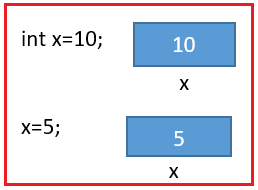Now in real-time programming, there will be a scenario where we need to store a group of values. You did not get it, right? Yeah, let us think this way. I want to store employee number of 10 employees. Then without an array, it is like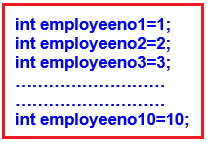I know you already feel this awkward. Yes, if the array kind of data structure is not there then programming would be a bit more complex. For everything we need to define a new variable even if it is of the same type. But let us see how the array solves this problem. We will discuss the syntax; the rules of declaring and initializing the array later part of this article in detail. For now, keep the focus on the concept only.

int[] employeeno = { 1, 2, 3, 4, 5};

##### How does using [] this work in real memory?

int[] empno = { 1, 2, 3, 4, 5 };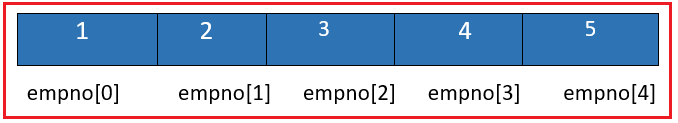See using this [] after the data type, you are informing the compiler that the variable is an array and allocating a block of memory as specified by the array.

##### Types of Arrays in C#:

C# supports 2 types of arrays. They are as follows:

1. Single dimensional array
2. Multi-dimensional array

In the Single dimensional array, the data is arranged in the form of a row whereas in the multi-dimensional arrays, the data is arranged in the form of rows and columns. Again, the multi-dimensional arrays are of two types

1. Jagged array: Whose rows and columns are not equal
2. Rectangular array: Whose rows and columns are equal

Note: We can access the values of an array using the index positions whereas the array index starts from 0 which means the first item of an array will be stored at the 0th position and the position of the last item of an array will be the total number of the item – 1. In this article, I am going to discuss how to work with Single Dimensional Array and in the next article, I am going to discuss the Multi-dimensional Array in C# with Examples.

##### How to declare an Array in C#?

We have discussed the importance of an array over normal variables but now let us discuss what are the different ways to declare an Array and initialize an Array in C# with examples.
General Syntax: <data type>[] VariableName = new <data type>[size of the array];
Example: int[] A = new int;

Here we have created an array with the name A and with a size of 5. So, you can store 5 values with the same name A. How it looks in the memory? It will allocate memory for 5 integers. These all 5 are types of int. For that memory, indexing will start from 0 onwards as shown in the below image.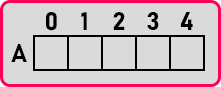We got an array. So, all these 5 integers are side by side or contiguous. Let us say the first byte’s address is 300 and let us assume int is taking 2 bytes then it will look like the below in the memory,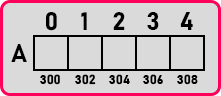So here A is taking 300-301. A is taking 302-303. A is taking 303-304 And so on. So how many bytes it is consuming in total? It will consume 10 bytes of memory. This is how we can create 5 variables with one name, so we say it is an array. The below is also an example of array declaration by specifying size by using a variable;

int size = 5;
int[] A = new int[size];

##### Declare and Initialize an Array in the Same Statement in C#

Just like declaring and initializing a normal variable in a single statement, we can also do the same for an array if we want to hardcode the input of the array; For example:

int[] A = { 1, 2, 3, 4, 5 };

##### How we can access the Array Elements in C#?

The answer is by using indexes. Let us proceed and try to understand how we can access the array elements using indexes.

##### What is an index of an array?

The index of an array is basically a pointer that is used to indicate which element in the array will be used. The array is sequential starting at zero to n-1, you can easily access any element in an array with the index. For example:

int[] empno={1,2,3,4,5};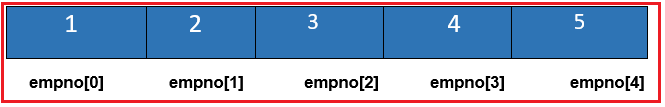In the above example to access the value four we can use the index 3 as follows:

Console.WriteLine(empno);

Note: The array index is an integer starting from 0. And the last index is the number of elements or array size -1. You can use the index to set and get the elements of an array in C#.

##### Memory Representation of Arrays in C#:

Please have a look at the following diagram: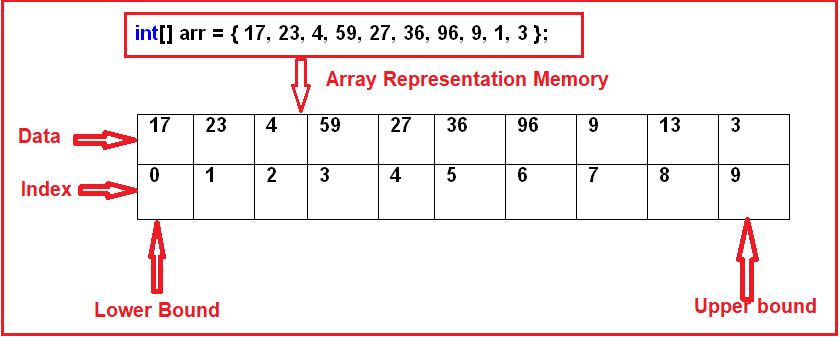As you can see in the above diagram, we have an integer array with 10 elements. The array index is starting from 0, which stores the first element of the array. As the array contains 10 elements, the last index position will be 9. The Array values or elements are stored sequentially in the memory i.e. contiguous memory location and this is the reason why it performs faster.

In C#, the arrays can be declared as fixed-length or dynamic. The Fixed length array means we can store a fixed number of elements while in the case of the dynamic array, the size of the array automatically increases as we add new items to the array. The Arrays in C# are reference types that are derived from the System.Array class.

##### Assigning Values to Array in C#:

By writing int[] n={1,2,3}; we are declaring and assigning values to the array at the same time, thus initializing it. But when we declare an array like int[] n = new int;, we need to assign values to it separately. Because int[] n = new int; will allocate space for 3 integers in the heap memory but there are no values in that space. To initialize it, assign a value to each of the elements of the array using its index position as shown below.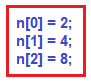It is just like we are declaring some variables and then assigning values to them as follows: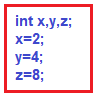Thus, the first way of assigning values to the elements of an array is by doing so at the time of its declaration i.e. int[] n={1,2,3}; And the second method is declaring the array first and then assigning values to its elements as shown below.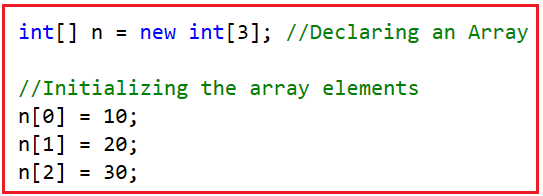You can understand this by treating n, n, and n as similar to the different variables you used before. Just like a variable, an array can be of any other data type also.

float[] f = new float; Here, ‘f’ is an array of floats.

##### How to Access Array Elements in C#?

Suppose we have an array as follows:
int[] n={1,2,3};

Now, you want to print 3 and you can see the value three is present at the index position 2, so to print the value 8, you need to use the array index position to 2 as follows:
Console.WriteLine(n);

So, we have to use the array name and the index for the value which we want to access. Now if we write,
Console.WriteLine(n); Will it print the whole array? No, we must print each and every element one by one separately. Or if you want to print all, then we can print them by using a loop such as for loop.

##### Can we use a for each loop to iterate on arrays in C#?

Yes. Since the arrays in C# are derived from the System.Array class which implements the IEnumerable, so we can use the for-each loop to iterate on arrays in C#.  We will see this practically once we start programming.

Now, I hope you understand what is an array, why we need an array, and how to create, initialize, and access the elements of an array in C#. Now, let us proceed and try to understand this concept in a better manner using some examples. In this article, we only keep focusing on Single-Dimensional Arrays, and in our next article, we will discuss Multi-Dimensional Arrays.

##### One Dimensional Array in C# with Examples:

The array which stores the data in the form of rows in a sequential order is called a one-dimensional array. The syntax for creating a one-dimensional array in C# is given below.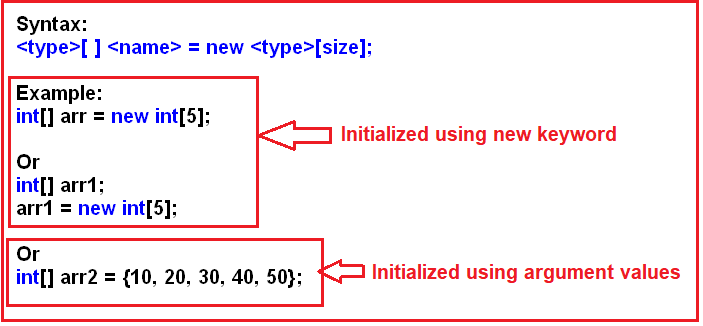As you can see in the above image, we can initialize an array in C# either by using the new keyword or by using the argument values. Using a new keyword, it is mandatory to specify the size and if you are initializing the array using the argument values, then based on the number of arguments, it will decide the size.

##### Example 1: Creating and Initializing an Array at the Same Statement

In the below example, we are creating an integer array with 3 elements. Then we are accessing the element individually using the array index position, then we are accessing the array element using a for loop, and also, we are accessing the array elements using a for each loop.

```using System;
namespace ArayDemo
{
class Program
{
static void Main(string[] args)
{
//Creating and Initializing an Array
//Here, the size will be decided based on the number of elements
//In this case size will be 3
int[] Numbers = { 10, 20, 30 };

//Accessing the Array Elements separately
Console.WriteLine("Accessing the Array Elements separately");
Console.WriteLine(\$"Numbers = {Numbers}");
Console.WriteLine(\$"Numbers = {Numbers}");
Console.WriteLine(\$"Numbers = {Numbers}");

//Accessing the Array Elements using for Loop
Console.WriteLine("\nAccessing the Array Elements using For Loop");
for (int i = 0; i <= Numbers.Length - 1; i++)
{
Console.WriteLine(\$"Numbers[{i}] = {Numbers[i]}");
}

//Accessing the Array Elements using foreach Loop
Console.WriteLine("\nAccessing the Array Elements using ForEach Loop");
foreach (int Number in Numbers)
{
Console.WriteLine(\$"{Number}");
}
}
}
}
```
###### Output: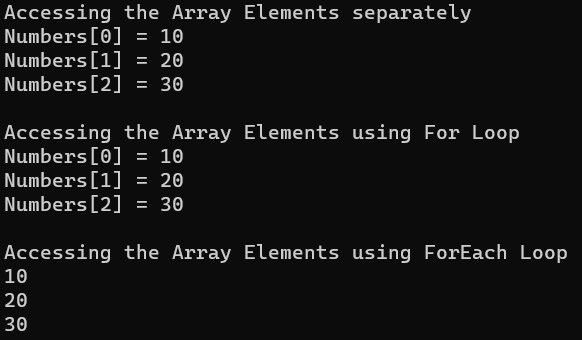##### Example 2: Creating and Initializing an Array Separately

In the below example, we are creating an integer array with size 3. That means this array can store a maximum of 3 integers. Without assigning any values to the memory location, we are printing the values to see what default values are stored. Then using the array index position, we are assigning values to the array and then print the values using a for loop.

```using System;
namespace ArayDemo
{
class Program
{
static void Main(string[] args)
{
//Creating an Integer Array with size 3
int[] Numbers = new int;

//Accessing the Array Elements Before Initialization
Console.WriteLine("Accessing the Array Elements Before Initialization");
for (int i = 0; i <= Numbers.Length - 1; i++)
{
Console.WriteLine(\$"Numbers[{i}] = {Numbers[i]}");
}

//Initializing the Array Elements using the Index Position
Numbers = 10;
Numbers = 20;
Numbers = 30;

//Accessing the Array Elements After Initialization
Console.WriteLine("\nAccessing the Array Elements After Initialization");
for (int i = 0; i <= Numbers.Length - 1; i++)
{
Console.WriteLine(\$"Numbers[{i}] = {Numbers[i]}");
}

}
}
}
```
###### Output: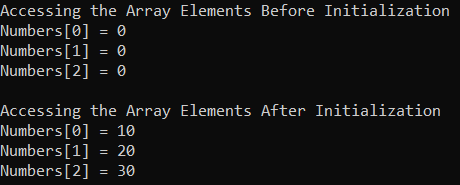##### Example 3: Dynamically Initializing an Array in C#

In the below example, first, we create an array with size 3. To check what default values an array in C# store, without initializing the array, we are printing the values on the console using a for loop. Then again, using a for loop we are assigning the elements to the array. Finally, we are accessing the array elements and printing the values on the console using a for each loop.

```using System;
namespace ArayDemo
{
class Program
{
static void Main(string[] args)
{
//Creating an array with size 3
int[] integerArray = new int;

//Accessing array values using loop
//Here it will display the default values
//as we are not assigning any values
for (int i = 0; i < integerArray.Length; i++)
{
Console.Write(integerArray[i] + " ");
}

Console.WriteLine();
int a = 0;

//Here we are assigning values to array using for loop
for (int i = 0; i < integerArray.Length; i++)
{
a += 10;
integerArray[i] = a;
}

//accessing array values using foreach loop
foreach (int i in integerArray)
{
Console.Write(i + " ");
}

}
}
}
```
###### Output: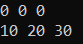As you can see in the above output, the default value 0, will store for integer type array. So far, in our examples, we have used a special loop called for each loop to access the array elements. Let us first understand what this for each loop is and then we will see the difference between for and for each loop in C#.

##### For Each Loop in C#:

The For Each loop is specially designed in C# for accessing the values from a collection like an Array, ArrayList, List, etc. When we use a for-each loop for accessing the values of an array or collection, we only require to hand over the array or collection to the loop which does not require any initialization, condition, or iteration. The loop itself starts its execution by providing access to each and every element present in the array or collection starting from the first up to the last element in sequential order.

##### What is the difference between for loop and for each loop in C# to access array values?

In the case of for loop in C#, the loop variable refers to the index of an array whereas, in the case of a for-each loop, the loop variable refers to the values of the array.

Irrespective of the values stored in the array, the loop variable must be of type int in case of for loop. The reason for this is, here the loop variable is referring to the index position of the array. Coming to the for-each loop, the data type of the loop variable must be the same as the type of the values stored in the array. For example, if you have a string array then the loop variable must be of type string in case of the for-each loop and int in case of a for loop in C#. For a better understanding, please have a look at the following example.

```using System;
namespace ArayDemo
{
class Program
{
static void Main(string[] args)
{
//Creating an array with size 3
string[] Countries = {"India", "USA", "UK" };

//Accessing array values using for loop
//Here, the loop variable is of integer type
for (int i = 0; i < Countries.Length; i++)
{
Console.Write(Countries[i] + " ");
}
Console.WriteLine();

//accessing array values using foreach loop
//Here, the loop variable is of type string
foreach (string country in Countries)
{
Console.Write(country + " ");
}

}
}
}
```

The most important point that you need to keep in mind is that the for loop in C# can be used both for accessing values from an array as well as assigning values to an array whereas the for-each loop in C# can only be used for accessing the values from an array but not for assigning values into an array.

##### What happens when you are trying to access an element whose index is out of bounds?

If you are trying to access an array whether for setting the value or getting the value, if that index is out of the bounds of an array, then it will throw an exception. For a better understanding, please have a look at the below example, here, we are creating an array whose size is 3 i.e. the index position is from 0 to 2 and we are trying to assign a value to the 3rd index position which does not exist. In this case, it will throw an exception.

```using System;
namespace ArayDemo
{
class Program
{
static void Main(string[] args)
{
//Creating an array with size 3
string[] Countries =new string;

Countries = "India";

}
}
}
```

It will not give you any compile time error, but when you run the above code, at runtime, it will throw the following exception.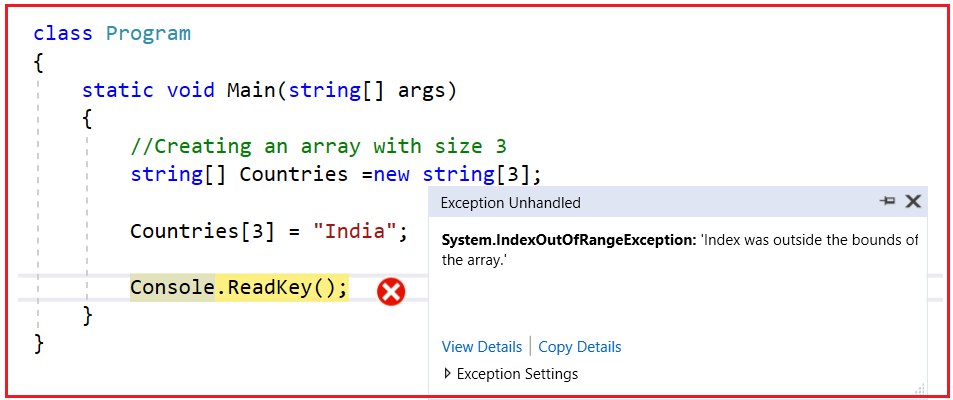##### Array Class in C#:

The Array class in C# is a predefined class that is defined inside the System namespaces. This class is working as the base class for all the Arrays in C#. The Array class provides a set of members (methods and properties) to work with the arrays such as creating, manipulating, searching, reversing, and sorting the elements of an array, etc. The definition of the Array class in C# is gen below.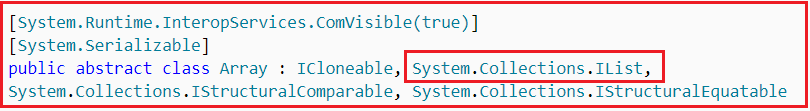The Array Class in C# is not a part of the System.Collections namespace. It is a part of the System namespace. But still, we considered it as a collection because it Implement the IList interface. The Array class provides the following methods and properties:

1. Sort(<array>)Sorting the array elements
2. Reverse (<array>)Reversing the array elements
3. Copy (src, dest, n)Copying some of the elements or all elements from the old array to the new array
4. GetLength(int)A 32-bit integer that represents the number of elements in the specified dimension.
5. LengthIt Returns the total number of elements in all the dimensions of the Array; zero if there are no elements in the array.
##### Example to Understand Array Class Methods and Properties in C#

Let us see an example for understanding the Method and Properties of the Array class in C#. The following example is self-explained, so please go through the comment lines.

```using System;
namespace ArayDemo
{
class Program
{
static void Main(string[] args)
{
//Creating and Initializing an Array of Integers
//Size of the Array is 10
int[] Numbers = { 17, 23, 4, 59, 27, 36, 96, 9, 1, 3 };

//Printing the Array Elements using a for Loop
Console.WriteLine("Original Array Elements :");
for (int i = 0; i < Numbers.Length; i++)
{
Console.Write(Numbers[i] + " ");
}
Console.WriteLine();

//Sorting the Array Elements by using the Sort method of Array Class
Array.Sort(Numbers);
//Printing the Array Elements After Sorting using a foreach loop
Console.WriteLine("\nArray Elements After Sorting :");
foreach (int i in Numbers)
{
Console.Write(i + " ");
}
Console.WriteLine();

//Reversing the array elements by using the Reverse method of Array Class
Array.Reverse(Numbers);
//Printing the Array Elements in Reverse Order
Console.WriteLine("\nArray Elements in the Reverse Order :");
foreach (int i in Numbers)
{
Console.Write(i + " ");
}
Console.WriteLine();

//Creating a New Array
int[] NewNumbers = new int;
//Copying Some of the Elements from Old array to new array
//We declare the array with size 10 and we copy only 5 elements.
//So the rest 5 elements will take the default value. In this array, it will take 0
Array.Copy(Numbers, NewNumbers, 5);

//Printing the Array Elements using for Each Loop
Console.WriteLine("\nNew Array Elements :");
foreach (int i in NewNumbers)
{
Console.Write(i + " ");
}

Console.WriteLine();
Console.WriteLine(\$"\nNew Array Length using Length Property :{NewNumbers.Length}");
Console.WriteLine(\$"New Array Length using GetLength Method :{NewNumbers.GetLength(0)}");
}
}
}
```
###### Output: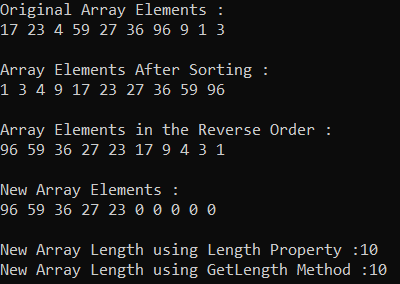##### Understanding the Implicitly Typed Arrays in C#:

When we declare an array by using the var keyword then such types of arrays are called implicitly typed arrays in C#.

Example: var arr = new[] {10, 20, 30 , 40, 50};

Let us see an example for a better understanding of the implicitly typed array in C#.

```using System;
namespace ArayDemo
{
class Program
{
static void Main(string[] args)
{
var Numbers = new[] { 10, 20, 30, 40, 50};
for (int i = 0; i < Numbers.Length; i++)
{
Console.Write(Numbers[i] + " ");
}
}
}
}
```

Output: 10 20 30 40 50

### 4 thoughts on “Arrays in C#”

1.its helpfull me a lot . thanks you so much

2.Your articles are perfect, helping me understand topics well, thank you.

3.Example1 output is invalid, Please Check

1.Hi, Thanks for letting us the same. We have updated the Example 1 output.